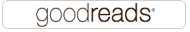Algorithms: a set of rules for solving a problem in a finite number of steps

# Algorithms

Algorithms: a set of rules for solving a problem in a finite number of steps1

## Resources

### BooksSome books on algorithms are rigorous but incomplete; others cover masses of material but lack rigor. Introduction to Algorithms uniquely combines rigor and comprehensiveness. The book covers a broad range of algorithms in depth, yet makes their design and analysis accessible to all levels of readers. Each chapter is relatively self-contained and can be used as a unit of study. The algorithms are described in English and in a pseudocode designed to be readable by anyone who has done a little programming. The explanations have been kept elementary without sacrificing depth of coverage or mathematical rigor.

### On-Line Courses

Introduction to Algorithms - This course provides an introduction to mathematical modeling of computational problems. It covers the common algorithms, algorithmic paradigms, and data structures used to solve these problems. The course emphasizes the relationship between algorithms and programming, and introduces basic performance measures and analysis techniques for these problems.

## Algorithmic Complexity

Algorithmic complexity is concerned about how fast or slow particular algorithm performs. We define complexity as a numerical function T(n) - time versus the input size n. We want to define time taken by an algorithm without depending on the implementation details. But you agree that T(n) does depend on the implementation! A given algorithm will take different amounts of time on the same inputs depending on such factors as: processor speed; instruction set, disk speed, brand of compiler and etc. The way around is to estimate efficiency of each algorithm asymptotically. We will measure time T(n) as the number of elementary "steps" (defined in any way), provided each such step takes constant time.2

• Time Complexity: Python - This page documents the time-complexity (aka "Big O" or "Big Oh") of various operations in current CPython. Other Python implementations (or older or still-under development versions of CPython) may have slightly different performance characteristics. 3
• A Gentle Introduction to Algorithm Complexity Analysis - However, theoretical computer science has its uses and applications and can turn out to be quite practical. In this article, targeted at programmers who know their art but who don't have any theoretical computer science background, I will present one of the most pragmatic tools of computer science: Big O notation and algorithm complexity analysis. As someone who has worked both in a computer science academic setting and in building production-level software in the industry, this is the tool I have found to be one of the truly useful ones in practice, so I hope after reading this article you can apply it in your own code to make it better. After reading this post, you should be able to understand all the common terms computer scientists use such as "big O", "asymptotic behavior" and "worst-case analysis".4

## Big O notation

Big O notation (with a capital letter O, not a zero), also called Landau's symbol, is a symbolism used in complexity theory, computer science, and mathematics to describe the asymptotic behavior of functions. Basically, it tells you how fast a function grows or declines. 5

• A beginner's guide to Big O notation - Big O notation is used in Computer Science to describe the performance or complexity of an algorithm. Big O specifically describes the worst-case scenario, and can be used to describe the execution time required or the space used (e.g. in memory or on disk) by an algorithm.
• Big O for Beginners - Big O Notation allows us to measure the time and space complexity of our code.

## Insertion Sort

This is an in-place comparison-based sorting algorithm. Here, a sub-list is maintained which is always sorted. For example, the lower part of an array is maintained to be sorted. An element which is to be 'insert'ed in this sorted sub-list, has to find its appropriate place and then it has to be inserted there. Hence the name, insertion sort.

The array is searched sequentially and unsorted items are moved and inserted into the sorted sub-list (in the same array). This algorithm is not suitable for large data sets as its average and worst case complexity are of Ο(n^2), where n is the number of items. 6

## Merge Sort

Merge sort is a sorting technique based on divide and conquer technique. With worst-case time complexity being Ο(n log n), it is one of the most respected algorithms.

Merge sort first divides the array into equal halves and then combines them in a sorted manner.7

Last edited by MichaelAlber .